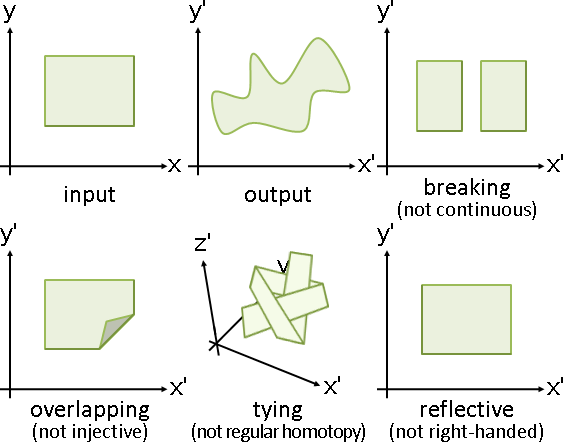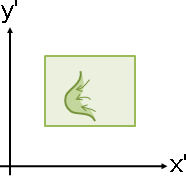Topological Surface🚧

Manifold（Topological Surface）

「流形」。伸縮自在的表面。

```set peoperty
1. closed/closure: only 1 connect component (and contain limit point)
2. bounded/boundary: cannot reach infinite
3. compact: closed + bounded

orientation:
1. mobius band
2. klein bottle
https://en.wikipedia.org/wiki/File:Flatsurfaces.svg

genus:
1. shpere = 0
2. torus = 1

embedding:
drawing
```
```gauss map / turning number / total curvature

gauss-bonnet theorem
1. closed curve   ∫Ω κ ds = 2π k
2. closed surface ∫M K dA = 2π χ(M)   where χ(M) = 2 - 2g

```

fundamental group

```1. fundamental group: basis of all cycles through a point
2. homotopy group: fundamental group generalization
3. homology group: non-degenerated cycles under homotopy???
4. #(fundamental group) = 2g
```
```tree-cotree decomposition [Eppstein 03]
spanning tree on planar graph / fundamental cycle basis
1. spanning tree T in primal graph
2. spanning cotree T* in dual graph (not cross edges of T)
3. for any edge not contained in T and not crossed by T*,
follow both of its vertices to the root T, a fundaemental cycle.

Euler characteristic χ = V - E + F = 2 - 2g
spanning tree T:    V-1 edges
spanning cotree T*: F-1 edges
remaining edges: E - (V-1) - (F-1) = -(2 - 2g) + 2 = 2g
```

orbifold

```orbifold / orientation
http://geometry.cs.cmu.edu/ddgshortcourse/AMSShortCourseDDG2018_DiscreteMappings.pdf
```

Surface Transformation🚧

Function

```數量：one-to-one, many-to-one, one-to-many
其中one-to-one又叫做injective

其中onto又叫做surjective

其中invertible又叫做bijective = injective + surjective
```

```鄰居：continuous

```

```相同相異：self-mapping, non-self-mapping
其中self-mapping又叫做autojective

其中global經常省略不寫
```

Function of Manifold

```態射morphism = continuous

https://math.stackexchange.com/questions/1672786/
```

```同倫homotopy = continuous

https://math.stackexchange.com/questions/296170/
```

local

```locally continuous injective is locally continuous bijective?
locally homeomoprhism is locally continuous and locally bijective.
locally bijective homeomorphism is globally bijective homeomorphism.
```

differentiable manifold

```derivative = Jacobian
immersion  = 輸出入先做derivative, the new mapping everywhere is injective
submersion = 輸出入先做derivative, the new mapping everywhere is surjective
```

harmonic manifold

```local bijection  iff  det(jacobian) > 0  everywhere
global bijection & convex  iff  coordinate is non-negative  everywhere
(diffeomorphism: X->Y is harmonic and Y is convex)
```

Surface Transformation🚧

Deformation（Topology-Preserving Transformation）

```不斷裂：Continuous        可逆不斷裂：Bicontinuous

``````一、連續函數的情況下：
一維：單射＝嚴格單調
高維：單射＝高維嚴格單調＝任意截面都是嚴格單調？

一維：單射＝雙射＝嚴格單調
高維：單射＝雙射＝高維嚴格單調＝任意截面都是嚴格單調？
```
```continuous injective = continuous strictly monotone
https://math.stackexchange.com/questions/279672/
https://math.stackexchange.com/questions/170147/

continuous injective self-mapping = continuous bijective
https://mathoverflow.net/questions/273632/
https://math.stackexchange.com/questions/541082/
```

```三、連續可微函數的情況下：
一維：單射＝嚴格單調＝處處斜率為正數、或者處處斜率為負數
高維：單射＝高維嚴格單調＝無奇點的和諧函數？

一維：單射＝雙射＝嚴格單調＝斜率處處正數或者處處負數
高維：單射＝雙射＝高維嚴格單調＝無奇點的和諧函數？
```
```continuous differentiable bijective = harmonic without singular point (?)
https://math.stackexchange.com/questions/61099/
```

```locally continuous：拓樸
locally differentiable：反函數定理
```「局部處處」與「全域」之間，已經發現許多關聯，也依然存在許多謎題。數學家目前只知道單向結論：

```四、連續可微自射函數的情況下：
高維：處處梯度可逆、且函數邊界數值趨近無限，導致全域雙射。
高維：處處梯度正定∇F≻0，導致全域單射。
高維：處處梯度對稱正定∇F=∇Fᵀ≻0，導致全域雙射。
高維：處處梯度行列式恆正det(∇F)>0，導致全域雙射？
```
```∇F is locally bijective everywhere and boundary(F) approaches ±∞ ⇒ globally bijective
https://math.stackexchange.com/questions/41551/

∇F is locally positive-definite everywhere ⇒ globally injective
https://math.stackexchange.com/questions/1820156/
https://math.stackexchange.com/questions/1372692/

∇F is locally symmetric positive-definite everywhere ⇒ globally bijective
https://math.stackexchange.com/questions/2874445/
```

Knot🚧

Knot

```Repulsive Curves
https://www.cs.cmu.edu/~kmcrane/
```

UVa 1624